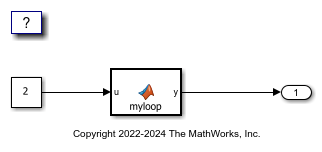# Unroll Parallel `for`-Loop That Has Small Number of Iterations

This example shows how to use the model configuration parameter Loop unrolling threshold to generate optimized code by unrolling parallel `for`-loops (`parfor`-loops). For a small number of loop iterations that perform some simple calculation, parallelization is inefficient as it introduces overhead, which includes time taken for thread creation, data synchronization between threads, and thread deletion. By unrollling small parallel `for`-loops, you can achieve improved execution speed. Unrolling loops that have a large number of iterations can significantly increase code generation time and generate inefficient code.

### Example Model

Open the example model `rtwdemo_unroll_parfor`. The model is configured to generate multithreaded code by selecting the Generate parallel for loops parameter.

```model = 'rtwdemo_unroll_parfor'; open_system(model) ```The MATLAB Function block contains this code:

```type('myloop.m') ```
```function y = myloop(u) y = ones(1,100); parfor (i = 1:5) y(i) = i+u; end end ```

The code contains a `parfor`-loop that has five iterations.

### Generate Code by Using the Default Threshold Value

1. Build the model.

```slbuild(model); ```
```### Starting build procedure for: rtwdemo_unroll_parfor ### Successful completion of build procedure for: rtwdemo_unroll_parfor Build Summary Top model targets built: Model Action Rebuild Reason ======================================================================================================= rtwdemo_unroll_parfor Code generated and compiled. Code generation information file does not exist. 1 of 1 models built (0 models already up to date) Build duration: 0h 0m 16.299s ```

Inspect the generated `rtwdemo_unroll_parfor_step` step function in the `rtwdemo_unroll_parfor.c` .

```file = fullfile('rtwdemo_unroll_parfor_ert_rtw','rtwdemo_unroll_parfor.c'); coder.example.extractLines(file,'/* Model step function */','/* Model initialize function',1,1); ```
```/* Model step function */ void rtwdemo_unroll_parfor_step(void) { int32_T i; int32_T i_0; /* MATLAB Function: '<Root>/MATLAB Function' */ for (i_0 = 0; i_0 < 100; i_0++) { rtwdemo_unroll_parfor_Y.Outport[i_0] = 1.0; } #pragma omp parallel for num_threads(omp_get_max_threads()) for (i = 0; i < 5; i++) { rtwdemo_unroll_parfor_Y.Outport[i] = ((real_T)i + 1.0) + 2.0; } /* End of MATLAB Function: '<Root>/MATLAB Function' */ } ```

The code generator generates a parallel `for`-loop for the MATLAB Function block because it has 5 iterations which is equal to the default threshold value.

### Generate Code by Raising the Threshold Value

1. Open the Configuration Parameters dialog box. On the Optimization pane, set the Loop unrolling threshold to `6` . Alternatively, use the command-line API:

```set_param(model, 'RollThreshold','6') ```

Build the model. Inspect the generated `rtwdemo_unroll_parfor_step` step function in the `rtwdemo_unroll_parfor.c`.

```slbuild(model); file = fullfile('rtwdemo_unroll_parfor_ert_rtw','rtwdemo_unroll_parfor.c'); coder.example.extractLines(file,'/* Model step function */','/* Model initialize function',1,1); ```
```### Starting build procedure for: rtwdemo_unroll_parfor ### Successful completion of build procedure for: rtwdemo_unroll_parfor Build Summary Top model targets built: Model Action Rebuild Reason ====================================================================================== rtwdemo_unroll_parfor Code generated and compiled. Generated code was out of date. 1 of 1 models built (0 models already up to date) Build duration: 0h 0m 12.866s /* Model step function */ void rtwdemo_unroll_parfor_step(void) { int32_T i; /* MATLAB Function: '<Root>/MATLAB Function' */ for (i = 0; i < 100; i++) { rtwdemo_unroll_parfor_Y.Outport[i] = 1.0; } rtwdemo_unroll_parfor_Y.Outport = 3.0; rtwdemo_unroll_parfor_Y.Outport = 4.0; rtwdemo_unroll_parfor_Y.Outport = 5.0; rtwdemo_unroll_parfor_Y.Outport = 6.0; rtwdemo_unroll_parfor_Y.Outport = 7.0; /* End of MATLAB Function: '<Root>/MATLAB Function' */ } ```

The code generator unrolls the parallel `for`-loop and produces a copy of the loop body for each iteration because it has fewer iterations compared to the threshold value.

### Clean Up Example Folders and Files

Close the model and remove temporary folders and files.

```bdclose(model); ```